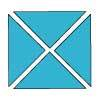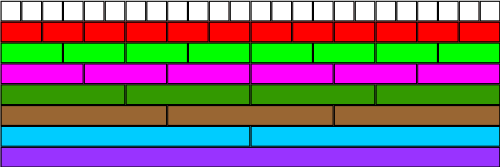#### You may also like### Chocolate

There are three tables in a room with blocks of chocolate on each. Where would be the best place for each child in the class to sit if they came in one at a time?### Four Triangles Puzzle

Cut four triangles from a square as shown in the picture. How many different shapes can you make by fitting the four triangles back together?### Cut it Out

Can you dissect an equilateral triangle into 6 smaller ones? What number of smaller equilateral triangles is it NOT possible to dissect a larger equilateral triangle into?

# Fractional Wall

##### Age 7 to 11Challenge Level

Thanks to the many of you who submitted a solution to this problem. Congratulations to Josie, Dominic and George from St Nicholas CE Junior School, Newbury and also Abigail from Histon Junior School and Charlie from Beckley C of E who all sent in clearly explained solutions. The solution given below was sent in by Cong:Using the image above, I can find $\frac{1}{2}$ as:
$1$ blue ($\frac{1}{2}$)
$2$ dark greens ($\frac{2}{4}$)
$3$ pinks ($\frac{3}{6}$)
$4$ light greens ($\frac{4}{8}$)
$6$ reds ($\frac{6}{12}$)
$12$ whites ($\frac{12}{24}$)

So I can also say that $$\frac{1}{2}= \frac{2}{4} = \frac{3}{6} = \frac{4}{8} = \frac{6}{12} = \frac{12}{24}$$

From the picture, I can find $\frac{1}{3}$ as:
$1$ brown ($\frac{1}{3}$)
$2$ pinks ($\frac{2}{6}$)
$4$ reds ($\frac{4}{12}$)
$8$ whites ($\frac{8}{24}$)

So I can also say that $$\frac{1}{3} = \frac{2}{6} = \frac{4}{12} = \frac{8}{24}$$

Again, using the image of the fraction wall, I can find $\frac{3}{4}$ as:
$3$ dark greens ($\frac{3}{4}$)
$6$ light greens ($\frac{6}{8}$)
$9$ reds ($\frac{9}{12}$)
$18$ whites ($\frac{18}{24}$)

So again I can say that $$\frac{3}{4}= \frac{6}{8} = \frac{9}{12} = \frac{18}{24}$$

The rule for working out equivalent fractions is to multiply the numerator and the denominator with the same whole number.